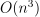# module `challenge.city_tour`#

## Short summary#

module `ensae_projects.challenge.city_tour`

Function to solve the problem of the Route Inspection Problem.

source on GitHub

## Classes#

class

truncated documentation

`SolutionException`

wrong solution

## Functions#

function

truncated documentation

`_delete_edge`

Removes an edge from the graph.

`_euler_path`

Computes an Eulerian path.

`_explore_path`

Explores an Eulerian path, remove used edges from edges_from.

`bellman_distances`

Computes shortest distances between all vertices. It assumes edges are symmetric.

`best_euler_path`

Computes the final solution for the Eulerian path.

`compute_degrees`

Compute the degree of vertices.

`dijkstra_path`

Returns the best path between two vertices. Uses Dikjstra

`distance_solution`

Checks if a solution is really a solution and returns the distance of it, None if it is not a solution. The function …

`distance_vertices`

Computes the length of edges if distances is None.

`euler_path`

Computes an Eulerian path.

`haversine_distance`

Computes Haversine formula.

`matching_vertices`

Finds the best match between vertices.

## Documentation#

Function to solve the problem of the Route Inspection Problem.

source on GitHub

exception ensae_projects.challenge.city_tour.SolutionException#

Bases: `Exception`

wrong solution

source on GitHub

ensae_projects.challenge.city_tour._delete_edge(edges_from, n, to)#

Removes an edge from the graph.

Parameters:
• edges_from – structure which contains the edges (will be modified)

• n – first vertex

• to – second vertex

Returns:

the edge

source on GitHub

ensae_projects.challenge.city_tour._euler_path(edges)#

Computes an Eulerian path.

Parameters:

edges – edges

Returns:

path, list of (vertex,edge)

The function assumes every vertex in the graph defined by edges has an even degree.

source on GitHub

ensae_projects.challenge.city_tour._explore_path(edges_from, begin)#

Explores an Eulerian path, remove used edges from edges_from.

Parameters:
• edges_from – structure which contains the edges (will be modified)

• begin – first vertex to use

Returns:

path

source on GitHub

ensae_projects.challenge.city_tour.bellman_distances(edges, distances, fLOG=None)#

Computes shortest distances between all vertices. It assumes edges are symmetric.

Parameters:
• edges – list of tuple (vertex A, vertex B)

• distances – distances (list of floats)

• fLOG – logging function

Returns:

dictionary of distances

This function could be implemented based on shortest_path.

source on GitHub

ensae_projects.challenge.city_tour.best_euler_path(edges, vertices, distances, edges_index=None, algo='blossom', fLOG=None)#

Computes the final solution for the Eulerian path.

Parameters:
• edges – edges (list of tuple)

• vertices – location of the vertices (not needed if distances are filled)

• distances – distances for each edge (list of distance)

• edges_index – list of indices of edges (if None –> range(len(edges))

• algo – algorithm to use to computes the matching (see `matching_vertices`)

• fLOG – logging function

Returns:

list of edges as tuple, list of edges as indices, distance

source on GitHub

ensae_projects.challenge.city_tour.compute_degrees(edges)#

Compute the degree of vertices.

Parameters:

edges – list of tuple

Returns:

dictionary {key: degree}

source on GitHub

ensae_projects.challenge.city_tour.dijkstra_path(edges, distances, va, vb)#

Returns the best path between two vertices. Uses Dikjstra algorithm.

Parameters:
• edges – list of edges.

• distances – list of distances

• va – first vertex

• vb – last vertex

Returns:

list of edges

This function could be implemented based on shortest_path.

source on GitHub

ensae_projects.challenge.city_tour.distance_solution(edges_index, edges, distances, solution, exc=True)#

Checks if a solution is really a solution and returns the distance of it, None if it is not a solution. The function does not case about the order.

Parameters:
• edges_index – list of indices of edges (if None –> range(len(edges))

• edges – list of tuple (vertex A, vertex B)

• distances – list of distances of each edge

• solution – proposed solutions (list of edge indices)

• exc – raises an exception in case of error

source on GitHub

ensae_projects.challenge.city_tour.distance_vertices(edges, vertices, distances)#

Computes the length of edges if distances is None.

Parameters:
• edges – list of tuple (vertex A, vertex B)

• vertices – locations of the vertices

• distances – distances (None or list of floats)

Returns:

distances (list of float)

source on GitHub

ensae_projects.challenge.city_tour.euler_path(edges_index, edges, solution)#

Computes an Eulerian path.

Parameters:
• edges_index – list of indices of edges (if None –> range(len(edges))

• edges – list of tuple (vertex A, vertex B)

• solution – proposed solutions (list of edge indices)

Returns:

path, list of edges indices

The function assumes every vertex in the graph defined by edges has an even degree.

source on GitHub

ensae_projects.challenge.city_tour.haversine_distance(lat1, lng1, lat2, lng2)#

Computes Haversine formula.

Parameters:
• lat1 – lattitude

• lng1 – longitude

• lat2 – lattitude

• lng2 – longitude

Returns:

distance

source on GitHub

ensae_projects.challenge.city_tour.matching_vertices(distances, algo='blossom')#

Finds the best match between vertices.

Parameters:
• distances – result of function @bellman_distances but only for odd vertices (odd = odd degree), dictionary { (vi,vj) : distance}

• algo – algorithm (see below)

Returns:

sequences of best matches.

If `algo=='hungarian'`, the function relies on linear_sum_assignment from scipy which uses the Hungarian Algorithm. Vertex index do not have to start from zero and be continuous. The function will handle that particular case. However, the method works for a bi-partite matching and is not suitable here unless the algorithm is modified. Not done (yet?).

If `algo=='blossom'`, it uses the Blossom algorithm which is known to be inand finds the optimal matching.

If `algo=='basic'`, the function sorts the distances by increasing order and builds new edges as they come in this list. It does not return an optimal solution but is much faster when the graph is big.

source on GitHub